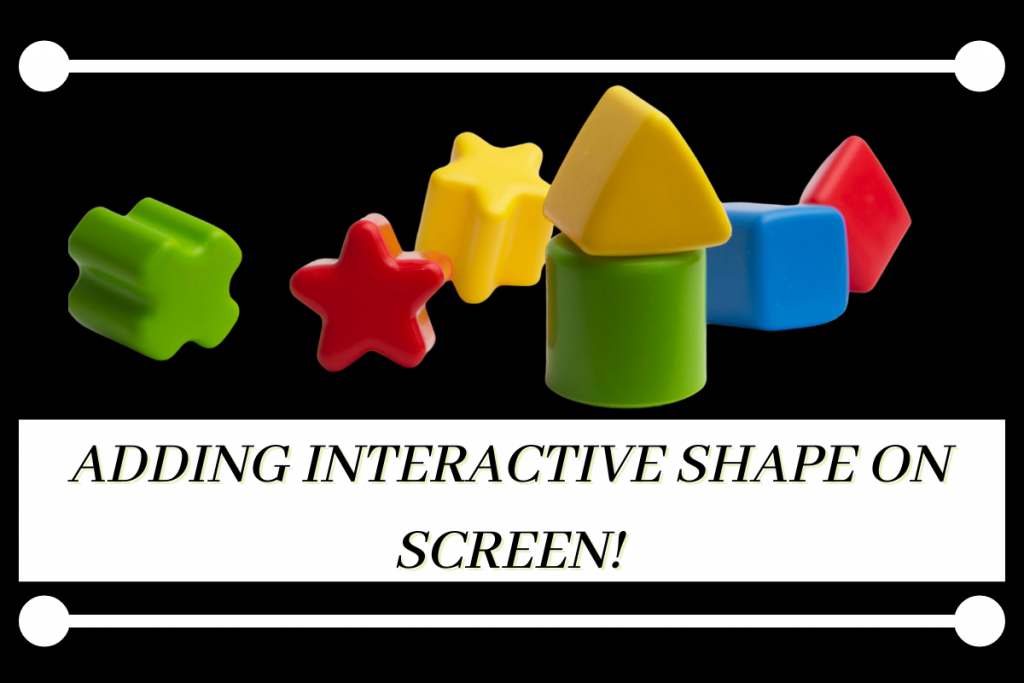# Pygame: Creating Interactive ShapesHey there! Today we are going to create a usual pygame screen but to spice things up we are going to add interactive shapes to it. Sounds interesting right?

So let’s begin!

## Step 1: Create a Basic Pygame Screen

Our first task is to create a pygame screen by first importing the necessary modules which include the `pygame` module, `pygame.locals` module. We also add the `mixer` module for the background music.

### 1. Creating a Window

We first initialize pygame and creating a window object by setting the height and width of the screen.

We will also add a running loop to handle various events like closing the window on pressing the `X` button.

### 2. Add a Background Image

The next thing we are going to do is add a background image by first loading the image and scaling the image in order to fill the whole window.

Then add the image by using the `blit` and `update` function inside the running loop.

### 3. Add the Background Music

We will be adding music into our program using the functions of the mixer module.

First we import the music from our folder of music files. For the same we use `music.load` function and then to play the music we use `music.play` function.

We will also be setting the volume of the music using the `music.set_volume` function.

The whole code for the designing of a basic customized screen as follows:

```import pygame
from pygame.locals import *
from pygame import mixer

pygame.init()
width = 500
height = 500
window = pygame.display.set_mode((width,height))
bg_img = pygame.transform.scale(bg_img,(width,height))

mixer.init()
mixer.music.load('Music File/Littleidea - wav music file.wav')
pygame.mixer.music.set_volume(0.05)
mixer.music.play()

runing = True
while runing:
window.blit(bg_img,(0,0))
for event in pygame.event.get():
if event.type == QUIT:
runing = False
pygame.display.update()
pygame.quit()
```

## Step 2: Add a Square on the Screen

To draw a Square we use the `draw.rect` function which takes three things: The window object name, the color of the rectangle and the dimentions of the rectangle ( width and height, x and y coordinates ).

We will define the width and height of the block before the running loop. And along with that we will declare the color of the block as well.

The code with the required code lines added is shown below. The changes made are highlighted for your reference.

```import pygame
from pygame.locals import *
from pygame import mixer
pygame.init()
width = 500
height = 500
window = pygame.display.set_mode((width,height))
bg_img = pygame.transform.scale(bg_img,(width,height))

x=y=50
color = "red"

mixer.init()
mixer.music.load('Music File/Littleidea - wav music file.wav')
pygame.mixer.music.set_volume(0.05)
mixer.music.play()
runing = True
while runing:
window.blit(bg_img,(0,0))
for event in pygame.event.get():
if event.type == QUIT:
runing = False

pygame.draw.rect(window, color, pygame.Rect(x, y, 60, 60))

pygame.display.update()
pygame.quit()
```

## Step 3: Add interactivity to the Square

Now to make the square in the following ways:

1. Up arrow key: Decrease y coordinate by 2
2. Down arrow key: Increase y coordinate by 2
3. Left arrow key: Decrease x coordinate by 2
4. Right arrow key: Increase x coordinate by 2

But before adding the arithmetic operations. We will make sure we capture the key that is being pressed using the `key.get_pressed` function and store it in a variable.

Then we will check the variable and apply the necessary changes in the coordinates based on the key captured.

The code lines to do the same is shown below:

```key = pygame.key.get_pressed()
if key[pygame.K_UP]:
y -= 2
if key[pygame.K_DOWN]:
y += 2
if key[pygame.K_LEFT]:
x -= 2
if key[pygame.K_RIGHT]:
x += 2
```

## Complete Implementation of Interactive Shapes in Pygame

The code below shows the final finished code. Hope you understood everything.

```import pygame
from pygame.locals import *
from pygame import mixer

pygame.init()

#window attributes
width = 500
height = 500
window = pygame.display.set_mode((width,height))
bg_img = pygame.transform.scale(bg_img,(width,height))

#square attributes
x=y=50
color = "red"

mixer.init()
mixer.music.load('Music File/Littleidea - wav music file.wav')
pygame.mixer.music.set_volume(0.05)
mixer.music.play()

#the running loop
runing = True
while runing:

window.blit(bg_img,(0,0))

#handling events
for event in pygame.event.get():
#closing window function
if event.type == QUIT:
runing = False

pygame.draw.rect(window, color, pygame.Rect(x, y, 60, 60))

#moving square on pressing keys
key = pygame.key.get_pressed()
if key[pygame.K_UP]:
y -= 2
if key[pygame.K_DOWN]:
y += 2
if key[pygame.K_LEFT]:
x -= 2
if key[pygame.K_RIGHT]:
x += 2

#update display
pygame.display.update()

#quit pygame
pygame.quit()
```

## The Final Output

The video below displays the final output of the code above. You can see how perfectly the square moves as the arrow keys are pressed!

## Conclusion

I hope this basic interactive shape tutorial helped you to learn something new in pygame!